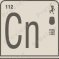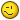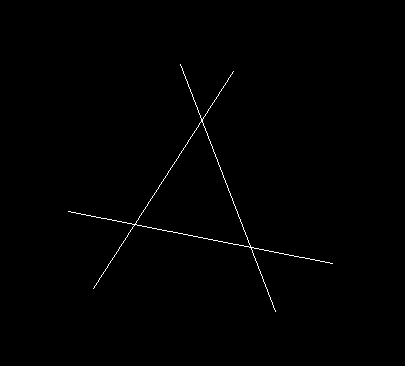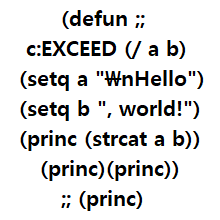# Required Lisp for area calculation.## Recommended PostsHi, everyone.

I am looking for a lisp command which can do the following.

1- Calculate area of enclosed area, the lines are not joint, but they are making enclosed area. When I click in side that area it should detect the lines and calculate area.

2- Then it should place an Alphabet their inside the enclosed area in capital form, when all 26 letters are placed it should go on with AA, AB, AC so on and so forth.

3- After clicking inside area and calculating the area, User should be able to place the label (value) by clicking in the cell of an already placed table as Field along with the Alphabet in the adjacent cell.

I am also thinking of other tools which can help in increasing the efficiency of my work.

Looking forward for positive replies from this forum.

thanks

##### Share on other sitesI have a program that does this. It also adds each closed polyline area and tag into a sql database that be queried. It can then display it on a website.

Sure it cost \$250k to buy and install and costs us about \$25K annually.It's called Archibus.

Look over at leemac's website to see what he has...

http://www.lee-mac.com/arealabel.html

•2
##### Share on other sitesPosted (edited)

1 Use a command called bpoly.

2 Has been answered before A-Z then AA -AZ I think it may have been here or forums/autodesk.

3 Because you have Lines a field option may not be possible, put ref & Area in table yes if change area  could do a update table. Again do Google.

Edited by BIGAL
##### Share on other sites##### Share on other sites1- Calculate area of enclosed area, the lines are not joint, but they are making enclosed area. When I click in side that area it should detect the lines and calculate area.

This doesn't make much sense. If the area is enclosed then the lines MUST be joined together, otherwise it can't be called an enclosed area.

But to answer your question, in AutoCAD simply select the polygon and type 'list' in command line and Bob's Yer Uncle##### Share on other sitesPosted (edited)
1 hour ago, psychopomp1 said:

This doesn't make much sense. If the area is enclosed then the lines MUST be joined together, otherwise it can't be called an enclosed area.

I guess they are talking about something like this. lines that cross but wouldn't make a polyline if you used the join command.Edited by mhupp
##### Share on other sitesWhy not use the Lee Mac lisp f700es posted for labeling the areas then use TCOUNT (Express Tool) to add letters as a prefix or suffix to the areas?

##### Share on other sites10 hours ago, psychopomp1 said:

This doesn't make much sense. If the area is enclosed then the lines MUST be joined together, otherwise it can't be called an enclosed area.

But to answer your question, in AutoCAD simply select the polygon and type 'list' in command line and Bob's Yer UncleBy joint I meant it is not a region or polyline or rectangle. Each line is separate entity, like lines are. Majority of the tools first ask to convert or create joint entities like rec or region to give area.
I have large and complex drawings . So going on and creating each area lines into those entities or calculating them takes a lot of time.

Also have tried many other methods which really are not that helpful.
So came up with this message so that if I can find any such lisp or tools then it would save a ton of time.

I hope this clarifies things a bit.

##### Share on other sites##### Share on other sitesI thought I posted a comment, like tombu's image you can use BPOLY to make a boundary inside a closed shape.

A slight different approach 2 stages just pick inside each area and label A,B C etc then ssget all these text loop through and do bpoly based on insertion point of text and get area. Either keep bpoly or erase.

I know I have done this method just quickly put this together to show how.

If you Google there is code for A-Z then AA -AZ etc.

```(defun c:test ( / lay ss txt txtstr txtins bp parea)
(setq lay (cdr (assoc 8 (entget (car (entsel "\nPick a text for layer "))))))
(setq ss (ssget "X" (list (cons 0 "*text")(cons 8 lay))))
(repeat (setq x (sslength ss))
(setq txt (vlax-ename->vla-object (ssname ss (setq x (- x 1)))))
(setq txtstr (vla-get-textstring txt))
(setq txtins (vlax-safearray->list (vlax-variant-value (vla-get-insertionpoint txt))))
(command "bpoly" txtins "")
(setq bp (entlast))
(setq parea (vla-get-area (vlax-ename->vla-object bp)))
(command "erase" bp "")
(princ (strcat "\nArea = " (rtos parea 2 3) " ID " txtstr))
)
(princ)
)```

##### Share on other sitesPosted (edited)

Looking at this in parts, not sure how stuck you are with this you see, but be patient with me.

So objective 1, getting the area and a slightly unusual method I think based on an answer to another question,  using '-Hatch' and picking an internal point:

Here you select a point enclosed by lines, polylines, circles, etc so long as the area is completely enclosed. Lines can be at different elevations I think. Exactly the same rules as if you were creating a hatch. You also need to see some of border for the area you want to calculate.

```(defun c:getarea ( / MyPt eo areA)
(setq MyPt (getpoint "Select Internal Point"))
(command "-hatch" MyPt "")
(setq obj (vlax-ename->vla-object (entlast)))
(setq area (vlax-get obj 'Area))
(entdel (entlast))

;;;;;;;;;;;;;;;;;;;;;;;;;;;;;
;;The above gets the area (as variable area, I'm a simple man) and a point (MyPt, nearly as obvious, that's a complex as I want to be)
;;Use the variable area as you want, here it is writng that to the point you selected initially, MyPt
(command "text" MyPt "" "" (strcat "Area = " (rtos area 2 2) " sq. units") "")

;;And here it is writing the area identifier, for example 'A' in this case
(setq MyPt (mapcar '+ '(0 5 0) MyPt))
(command "text" MyPt "" "" "A" "")

(princ)
)```

Used in a loop you could ask the user to enter or select the most recently used reference text (A, B, AA, AB, or whatever), increment that by 1, ask the user to select a point in the area and then loop to select a point in the next area saving or select the table cells and then loop as your preference might be. I would prefer to select all the areas, save their areas into a list and label them before going to the table to complete that.

For the next stage, have you got an example table you would use that could be used to check out populating what you want and how?

Edited by Steven P
•1
##### Share on other sitesThis is something I have wanted to do for a while, felt inspired to do it this evening and is stage 2of the question I think incrementing the area references (A ->B etc).

Written to replace what I was using though some notes still in there for things to finish off at another date. The Command (xyzuprev Txt inc) where Txt is the text string to increase by the number, 'inc'. Will increase numbers (including leading zeros), and letter or combinations of them

Will fail if 'inc' is more than 9 or 26 (for numbers / letters) - just me being lazy to not correct that yet, but for this question that is good enough

(setq AreaLabel (getstring "\nEnter First Area Label: ")) ;;as the 2nd line

and

(setq inc 1)
(setq AreaLabel (xyzuprev AreaLabel inc)) ;;as the last 2 lines in the while loop

to the code above to get it to work

```(defun xyzuprev (Txt inc / TxtCount SplitTxt IncTextList )
;; 0: Ascii 48 ;; 9: Ascii 57
;; A: ascii 65 ;; Z: Ascii 90
;; a: ascii 97 ;; z: Ascii 122
;;; Sub Functions ;;;;
;;https://forums.autodesk.com/t5/visual-lisp-autolisp-and-general/lisp-replace-an-item/td-p/10768078
(defun plusp (num) (cond ((numberp num) (>= num 0.0))))
(defun set_nth (lst n value) ;Sets n-th element of a list to new value (set_nth '(1 2 3 4) 2 5) -> (1 2 5 4)
(cond
((and (plusp n) (<= n (length lst)))
(cond
((zerop n)
(cons value (cdr lst))
)
(t (cons (car lst) (set_nth  (cdr lst) (1- n) value)))
))))
(defun txt2list( Txt / SplitTxt acount) ;;seperate characters to list items
(setq SplitTxt (list) )
(setq acount 0)
(while (< acount (strlen Txt))
(setq SplitTxt (append SplitTxt (list(substr Txt (+ acount 1) 1))))
(setq acount (+ acount 1))
) ;end while
SplitTxt
)
(defun list2txt( SplitTxt / Txt acount) ;;Join list items to a text string
(setq acount 0)
(setq Txt "")
(while (< acount (length SplitTxt))
(setq Txt (strcat Txt (nth acount SplitTxt)))
(setq acount (+ acount 1))
) ;end while
Txt
)
(defun inclisttext ( Lst Pos Inc / nextinc ) ;increment a text character in a string
(setq nextinc 0)
;; Add here error check if the list item is more than 1 character long
(setq Units (+ (ascii (nth Pos Lst)) Inc)) ;; ASCII CODE
;; these should be conds really
(if (and (< 57 Units)(> 65 Units)) (progn
(setq Units (- Units 10))
(setq nextinc 1)
))
(if (and (< 90 Units)(> 97 Units)) (progn
(setq Units (- Units 26))
(setq nextinc 1)
))
(if (< 122 Units) (progn
(setq Units (- Units 26))
(setq nextinc 1)
))
(list (set_nth Lst Pos (chr Units)) nextinc)
)
;;;; End Sub Functions ;;;;;

;; add here pause cmd echo
;; Add here check if text is a date

(if (numberp Txt)(rtos Txt)) ;; Make text a string
(setq TxtCount 0)
(while (< TxtCount (strlen Txt))
(setq SplitTxt (reverse (txt2list Txt)))
(setq IncTextList (inclisttext SplitTxt TxtCount inc))
(setq Txt (list2Txt (reverse (nth 0 IncTextList))) )
(setq inc (nth 1 IncTextList))
(setq TxtCount (+ TxtCount 1))
)
(if (= 1 inc)(alert "Text Length exceeded"))
;; add her a check if Txt was a number, return it to being a number
;; finish LISP, CMD echo, error, undo etc.
Txt
)```

•1
##### Share on other sitesSteven P have a look at this start with 1 = A then try 26 and 27 and so on.

```;-------------------------------------------------------------------------------
; Number2Alpha - Converts Number into Alpha string
; Function By: Gilles Chanteau from Marseille, France
; Arguments: 1
;   Num# = Number to convert
; Syntax example: (Number2Alpha 731) = "ABC"
;-------------------------------------------------------------------------------
(defun Number2Alpha (Num# / Val#)
(if (< Num# 27)
(chr (+ 64 Num#))
(if (= 0 (setq Val# (rem Num# 26)))
(strcat (Number2Alpha (1- (/ Num# 26))) "Z")
(strcat (Number2Alpha (/ Num# 26)) (chr (+ 64 Val#)))
)
)
);defun Number2Alpha```

(Number2Alpha 1)

(Number2Alpha 26)

(Number2Alpha 27)

(Number2Alpha 52)

•1
##### Share on other sites1 hour ago, BIGAL said:

Steven P have a look at this start with 1 = A then try 26 and 27 and so on.

```;-------------------------------------------------------------------------------
; Number2Alpha - Converts Number into Alpha string
; Function By: Gilles Chanteau from Marseille, France
; Arguments: 1
;   Num# = Number to convert
; Syntax example: (Number2Alpha 731) = "ABC"
;-------------------------------------------------------------------------------
(defun Number2Alpha (Num# / Val#)
(if (< Num# 27)
(chr (+ 64 Num#))
(if (= 0 (setq Val# (rem Num# 26)))
(strcat (Number2Alpha (1- (/ Num# 26))) "Z")
(strcat (Number2Alpha (/ Num# 26)) (chr (+ 64 Val#)))
)
)
);defun Number2Alpha```

(Number2Alpha 1)

(Number2Alpha 26)

(Number2Alpha 27)

(Number2Alpha 52)

some kind of magic?

##### Share on other sites14 hours ago, Steven P said:

This is something I have wanted to do for a while, felt inspired to do it this evening and is stage 2of the question I think incrementing the area references (A ->B etc).

Written to replace what I was using though some notes still in there for things to finish off at another date. The Command (xyzuprev Txt inc) where Txt is the text string to increase by the number, 'inc'. Will increase numbers (including leading zeros), and letter or combinations of them

Will fail if 'inc' is more than 9 or 26 (for numbers / letters) - just me being lazy to not correct that yet, but for this question that is good enough

(setq AreaLabel (getstring "\nEnter First Area Label: ")) ;;as the 2nd line

and

(setq inc 1)
(setq AreaLabel (xyzuprev AreaLabel inc)) ;;as the last 2 lines in the while loop

to the code above to get it to work

```(defun xyzuprev (Txt inc / TxtCount SplitTxt IncTextList )
;; 0: Ascii 48 ;; 9: Ascii 57
;; A: ascii 65 ;; Z: Ascii 90
;; a: ascii 97 ;; z: Ascii 122
;;; Sub Functions ;;;;
;;https://forums.autodesk.com/t5/visual-lisp-autolisp-and-general/lisp-replace-an-item/td-p/10768078
(defun plusp (num) (cond ((numberp num) (>= num 0.0))))
(defun set_nth (lst n value) ;Sets n-th element of a list to new value (set_nth '(1 2 3 4) 2 5) -> (1 2 5 4)
(cond
((and (plusp n) (<= n (length lst)))
(cond
((zerop n)
(cons value (cdr lst))
)
(t (cons (car lst) (set_nth  (cdr lst) (1- n) value)))
))))
(defun txt2list( Txt / SplitTxt acount) ;;seperate characters to list items
(setq SplitTxt (list) )
(setq acount 0)
(while (< acount (strlen Txt))
(setq SplitTxt (append SplitTxt (list(substr Txt (+ acount 1) 1))))
(setq acount (+ acount 1))
) ;end while
SplitTxt
)
(defun list2txt( SplitTxt / Txt acount) ;;Join list items to a text string
(setq acount 0)
(setq Txt "")
(while (< acount (length SplitTxt))
(setq Txt (strcat Txt (nth acount SplitTxt)))
(setq acount (+ acount 1))
) ;end while
Txt
)
(defun inclisttext ( Lst Pos Inc / nextinc ) ;increment a text character in a string
(setq nextinc 0)
;; Add here error check if the list item is more than 1 character long
(setq Units (+ (ascii (nth Pos Lst)) Inc)) ;; ASCII CODE
;; these should be conds really
(if (and (< 57 Units)(> 65 Units)) (progn
(setq Units (- Units 10))
(setq nextinc 1)
))
(if (and (< 90 Units)(> 97 Units)) (progn
(setq Units (- Units 26))
(setq nextinc 1)
))
(if (< 122 Units) (progn
(setq Units (- Units 26))
(setq nextinc 1)
))
(list (set_nth Lst Pos (chr Units)) nextinc)
)
;;;; End Sub Functions ;;;;;

;; add here pause cmd echo
;; Add here check if text is a date

(if (numberp Txt)(rtos Txt)) ;; Make text a string
(setq TxtCount 0)
(while (< TxtCount (strlen Txt))
(setq SplitTxt (reverse (txt2list Txt)))
(setq IncTextList (inclisttext SplitTxt TxtCount inc))
(setq Txt (list2Txt (reverse (nth 0 IncTextList))) )
(setq inc (nth 1 IncTextList))
(setq TxtCount (+ TxtCount 1))
)
(if (= 1 inc)(alert "Text Length exceeded"))
;; add her a check if Txt was a number, return it to being a number
;; finish LISP, CMD echo, error, undo etc.
Txt
)```

But I lost to Gilles, haha

It is super simple and correct,

it supports up to the limit of fix num, so the range is wider.

it's mathematically interesting. It has been a study for me.

Edited by exceed
•2
##### Share on other sitesYup, I saw that one the other day Exceed, for what I was wanting to do BigAls idea might be simpler. If I get chance this week though I will try your version too

•1
##### Share on other sitesWhen you just want A-Z its easy just use (chr 65) (chr 66).......  but is limited to A-Z, a-z (chr 97) .....

Credit to Gilles though look in getexcel for 2 more.

•1
##### Share on other sites12 hours ago, BIGAL said:

When you just want A-Z its easy just use (chr 65) (chr 66).......  but is limited to A-Z, a-z (chr 97) .....

Credit to Gilles though look in getexcel for 2 more.

Weird thoughts come at weird times but I was thinking last night as I did the dishes (Yes, I know....), it might not work so well with alpha-numeric numbers? (A1 etc where A9 -> A10), or a mix of types (caps and lower cases, aA)?

Might still have to loop through the text string to find if the last characters are numbers, all letters, or are upper or lower cases, would that be more efficient than looping through the text and incrementing as required.

##### Share on other sitesCheck out the code and A23 is one of the other functions.

```;-------------------------------------------------------------------------------
; ColumnRow - Returns a list of the Column and Row number
; Function By: Gilles Chanteau from Marseille, France
; Arguments: 1
;   Cell\$ = Cell ID
; Syntax example: (ColumnRow "ABC987") = '(731 987)
;-------------------------------------------------------------------------------
(defun ColumnRow (Cell\$ / Column\$ Char\$ Row#)
(setq Column\$ "")
(while (< 64 (ascii (setq Char\$ (strcase (substr Cell\$ 1 1)))) 91)
(setq Column\$ (strcat Column\$ Char\$)
Cell\$ (substr Cell\$ 2)
);setq
);while
(if (and (/= Column\$ "") (numberp (setq Row# (read Cell\$))))
(list (Alpha2Number Column\$) Row#)
'(1 1);default to "A1" if there's a problem
);if
);defun ColumnRow
;-------------------------------------------------------------------------------
; Alpha2Number - Converts Alpha string into Number
; Function By: Gilles Chanteau from Marseille, France
; Arguments: 1
;   Str\$ = String to convert
; Syntax example: (Alpha2Number "ABC") = 731
;-------------------------------------------------------------------------------
(defun Alpha2Number (Str\$ / Num#)
(if (= 0 (setq Num# (strlen Str\$)))
0
(+ (* (- (ascii (strcase (substr Str\$ 1 1))) 64) (expt 26 (1- Num#)))
(Alpha2Number (substr Str\$ 2))
);+
);if
);defun Alpha2Number
;-------------------------------------------------------------------------------
; Number2Alpha - Converts Number into Alpha string
; Function By: Gilles Chanteau from Marseille, France
; Arguments: 1
;   Num# = Number to convert
; Syntax example: (Number2Alpha 731) = "ABC"
;-------------------------------------------------------------------------------
(defun Number2Alpha (Num# / Val#)
(if (< Num# 27)
(chr (+ 64 Num#))
(if (= 0 (setq Val# (rem Num# 26)))
(strcat (Number2Alpha (1- (/ Num# 26))) "Z")
(strcat (Number2Alpha (/ Num# 26)) (chr (+ 64 Val#)))
);if
);if
);defun Number2Alpha```

•1

## Join the conversation

You can post now and register later. If you have an account, sign in now to post with your account.
Note: Your post will require moderator approval before it will be visible.×   Pasted as rich text.   Restore formatting

Only 75 emoji are allowed.